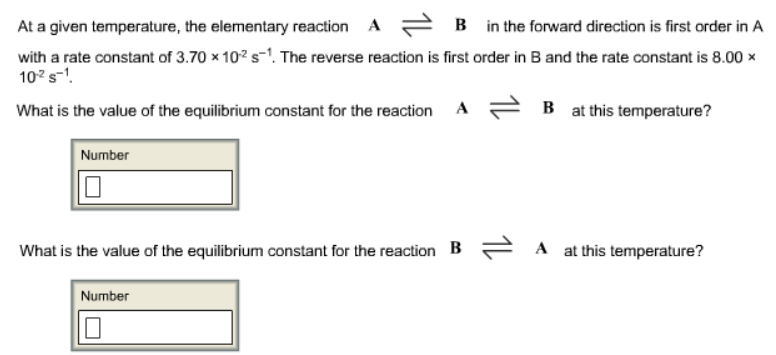# Problem: At a given temperature, the elementary reaction A ⇌ B in the forward direction is first order in A with a rate constant of 3.70 x 10-2 s-1. The reverse reaction is first order in B and the rate constant is 8.00 x 10-2 s-1. What is the value of the equilibrium constant for the reaction A ⇌ B at this temperature? What is the value of the equilibrium constant for the reaction B ⇌ A at this temperature?

###### FREE Expert Solution
97% (337 ratings)###### Problem Details

At a given temperature, the elementary reaction A ⇌ B in the forward direction is first order in A with a rate constant of 3.70 x 10-2 s-1. The reverse reaction is first order in B and the rate constant is 8.00 x 10-2 s-1

What is the value of the equilibrium constant for the reaction A ⇌ B at this temperature?

What is the value of the equilibrium constant for the reaction B ⇌ A at this temperature?